## 20111230

### Astronomy haiku: dark matter

Astronomy 210 Haiku review, fall semester 2011
Cuesta College, San Luis Obispo, CA

Students find their assigned groups of three to four students, and work cooperatively on an worksheet to review for a midterm.

Identify the relevant astronomy concept/answer for this haiku. Briefly explain your choice. Partial credit possible.
Surrounding halo,
invisible gravity.
Holds Milky Way tight.
Concept: __________
Explanation: __________

Section 70160
"Dark matter--Evidence that extra mass lies in extended halo sometimes called dark halo. Not luminous matter detected by its gravitational force."

"Dark matter--No one has seen dark matter because it doesn't give off light, but it creates gravity, so we know it exists."

"Dark matter--Proves Kepler's third law wrong because stars farther out do not move slower because there is no center of gravity."

"Dark matter--Dark matter is gravity that holds the Milky Way together and is unable to be seen."

"Dark matter--The Milky Way doesn't follow Kepler's third law."

"Dark matter--Dark matter is invisible and it holds everything inside the Milky Way."

"Dark matter--Dark matter holds the Milky Way together."
Section 70158
"Dark matter--That's what holds the Milky Way together."

"Monolithic collapse--flattens out over time with halo making disk left."

"Dark matter--Dark matter is the unseen gravity force which fills the universe around galaxies, surrounding the Milky Way."

"Black hole! You know black holes are present when there is a gravity alteration like an entire galaxy clumped together."

"Dark matter--Dark matter is the stuff that surrounds the Milky Way and keeps everything tight."

"Black hole--Black holes are at the center of spiral galaxies and have infinite gravity due to their immense density."

"Dark matter--Dark matter surrounds galaxies, holding them together as they rotate with lots of mass."

"Antimatter/dark matter--is found all around the Milky Way and is unexplained but we know it's there."

Dark matter--Dark matter is invisible but still creates a gravitational pull."

## 20111228

### Astronomy in-class activity: planet-hunting

Astronomy 210 In-class activity 6 v.11.12.22, spring semester 2012
Cuesta College, San Luis Obispo, CA

Students find their assigned groups of three to four students, and work cooperatively on an in-class activity worksheet to determine where in the sky each naked-eye planet will be observed on a given date (here, February 2, 2012).Previous posts:

## 20111227

### Astronomy final exam question: apparent magnitudes replaced with absolute magnitudes?

Astronomy 210 Final Exam, fall semester 2011
Cuesta College, San Luis Obispo, CA

An astronomy question on an online discussion board[*] was asked and answered:
Pdg: If the apparent magnitude of every star in our night sky were changed to its absolute magnitude value what would the night sky look like then?
tham153: Because most stars are further away than 10 parsecs, the night sky would be brighter.
Discuss whether or not if this answer is correct, and how you know this. Explain using the properties of apparent magnitude, absolute magnitude, and distances to stars.

• p:
Correct. Understands difference between apparent magnitude m values and absolute magnitude M values, and that stars closer or farther than 10 parsecs away will appear dimmer or brighter, respectively, if apparent magnitudes were replaced with absolute magnitude values. May argue night sky will be brighter assuming this is done to all stars in Milky Way (or universe), or may argue night sky will be dimmer assuming that this is done only to (visible?) stars in night sky.
• r:
Nearly correct (explanation weak, unclear or only nearly complete); includes extraneous/tangential information; or has minor errors.
• t:
Contains right ideas, but discussion is unclear/incomplete or contains major errors. At least understands the difference between m and M values with respect to star distances, and that smaller positive (or more negative) magnitudes are brighter.
• v:
Limited relevant discussion of supporting evidence of at least some merit, but in an inconsistent or unclear manner. Garbled definitions/relations between d, m, and M.
• x:
Implementation/application of ideas, but credit given for effort rather than merit.
• y:
Irrelevant discussion/effectively blank.
• z:
Blank.
Exam code: finalsf4R
p: 15 students
r: 2 students
t: 2 students
v: 13 students
x: 0 students
y: 0 students
z: 1 student

Exam code: finalN3aR
p: 16 students
r: 2 students
t: 3 students
v: 0 students
x: 2 students
y: 0 students
z: 0 students

A sample "p" response (from student 1985):Another sample "p" response (from student 6288):A sample "v" response (from student 8436), claiming "SWAG" (a scientific wild-a## guess):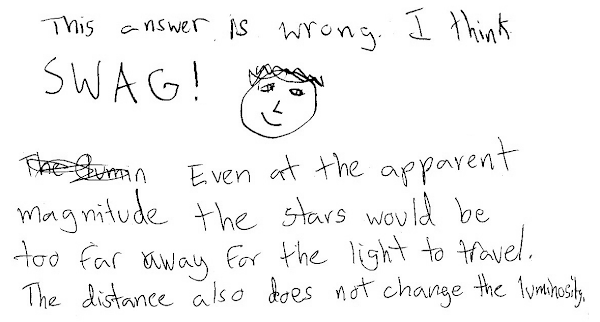## 20111226

### Astronomy final exam question: why Mars inhospitable?

Astronomy 210 Final Exam, fall semester 2011
Cuesta College, San Luis Obispo, CA

An astronomy question on an online discussion board(*) was asked and answered:
CARTART: Why is Mars [too] cold to support life?
yeah: [B]ecause [M]ars is farther from the sun.
swbarnes2: Another factor for Mars is...its small mass...
Discuss why the answers from "yeah" and "swbarnes2" both correctly answer the question from "CARTART," and how you know this. Explain using the properties of greenhouse gases and geological activity.

• p = 20/20:
Correct. Discusses answers from both "yeah" and "swbarnes2." Being farther from the sun, Mars would receive less radiation for a greenhouse effect. Mars' small mass would mean less outgassing, and also be unable to retain an atmosphere sufficient for greenhouse effect.
• r = 16/20:
Nearly correct (explanation weak, unclear or only nearly complete); includes extraneous/tangential information; or has minor errors. One of two explanations is problematic/incomplete, the other is correct.
• t = 12/20:
Contains right ideas, but discussion is unclear/incomplete or contains major errors. Both answers are problematic/incomplete, or one answer correct, while the other is missing.
• v = 8/20:
Limited relevant discussion of supporting evidence of at least some merit, but in an inconsistent or unclear manner. At least understands factors that contribute to atmosphere density and greenhouse effect.
• x = 4/20:
Implementation/application of ideas, but credit given for effort rather than merit. Discusses factors other than relevant to the greenhouse effect.
• y = 2/20:
Irrelevant discussion/effectively blank.
• z = 0/20:
Blank.
Exam code: finalsf4R
p: 13 students
r: 4 students
t: 5 students
v: 7 students
x: 3 students
y: 0 students
z: 1 student

Exam code: finalN3aR
p: 9 students
r: 6 students
t: 5 students
v: 5 students
x: 1 student
y: 0 students
z: 0 students

A sample "p" response (from student 2286):A sample "p" response (from student 2003):## 20111224

### Student scribble: "dwarf planet"Doodle by E. Winkle. Astronomy 210 Final Exam, Cuesta College, San Luis Obispo, CA.

## 20111222

### Physics final exam question: cooling a liquid-filled glass

Physics 205A Final Exam, fall semester 2011
Cuesta College, San Luis Obispo, CA

Cf. Giambattista/Richardson/Richardson, Physics, 2/e, Problems 13.14, 13.15

A glass container is filled to the brim with a liquid when the system is at 40° C. After the system is cooled to 15° C, the liquid level is slightly below the top of the container. There are no phase changes. Which material (glass or liquid) has a larger coefficient of volume expansion? Explain your answer using the properties of temperature and thermal expansion.

• p:
Correct. Argues that since liquid contracted more than the glass, then the liquid must have a greater volume expansion coefficient than the glass.
• r:
As (p), but argument indirectly, weakly, or only by definition supports the statement to be proven, or has minor inconsistencies or loopholes.
• t:
Nearly correct, but argument has conceptual errors, or is incomplete.
• v:
Limited relevant discussion of supporting evidence of at least some merit, but in an inconsistent or unclear manner.
• x:
Implementation of ideas, but credit given for effort rather than merit.
y: Irrelevant discussion/effectively blank.
• y:
Irrelevant discussion/effectively blank.
• z:
Blank.

Sections 70854, 70855
Exam code: finalB0xs
p: 37 students
r: 8 students
t: 0 students
v: 1 student
x: 1 student
y: 1 student
z: 0 students

A sample "p" response (from student 3737):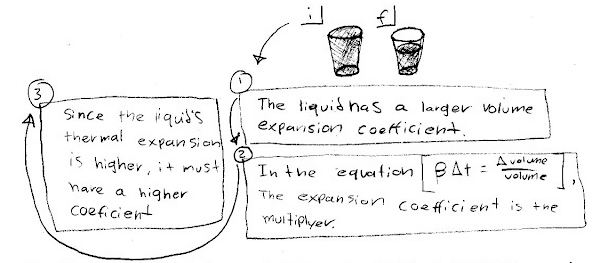A sample "p" response (from student 4242):## 20111221

### Physics final exam question: temperature changes during heat exchange

Physics 205A Final Exam, fall semester 2011
Cuesta College, San Luis Obispo, CA

Cf. Giambattista/Richardson/Richardson, Physics, 2/e, Practice Problem 14.5, Comprehensive Problem 14.75

A 0.50 kg aluminum sample at a temperature of 25° C, and a 0.50 kg iron sample at a temperature of 50° C are brought together in contact to reach thermal equilibrium. After reaching thermal equilibrium, which sample had the greatest change in temperature? Show your work and explain your reasoning using the properties of heat, temperature, and thermal equilibrium. Specific heat of aluminum is 900 J/(kg·K). Specific heat of iron is 440 J/(kg·K).

• p:
Correct. The heat transferred from the iron sample is equal to the heat transferred to the aluminum sample as they attain thermal equilibrium. Because they have the same mass, then the iron sample, which has the smaller specific heat, will have the greater temperature change. May have explicitly solved for the final temperature of the aluminum-iron system.
• r:
As (p), but argument indirectly, weakly, or only by definition supports the statement to be proven, or has minor inconsistencies or loopholes. Recognizes that iron has a smaller specific heat in arguing that it must have the greater temperature change, but does not explicitly note that the heat or change in internal energy is the same for both samples as they attain thermal equilibrium.
• t:
Nearly correct, but argument has conceptual errors, or is incomplete. At least discussion involves heat, specific heats, and temperature changes.
• v:
Limited relevant discussion of supporting evidence of at least some merit, but in an inconsistent or unclear manner.
• x:
Implementation of ideas, but credit given for effort rather than merit.
y: Irrelevant discussion/effectively blank.
• y:
Irrelevant discussion/effectively blank.
• z:
Blank.

Sections 70854, 70855
Exam code: finalB0xs
p: 20 students
r: 9 students
t: 16 students
v: 1 student
x: 1 student
y: 1 student
z: 0 students

A sample "p" response (from student 0864):Another sample "p" response (from student 1123):## 20111220

### Physics final exam problem: accelerating stacked boxes

Physics 205A Final Exam, fall semester 2011
Cuesta College, San Luis Obispo, CA

Cf. Giambattista/Richardson/Richardson, Physics, 2/e, Problems 4.142, 4.144Two boxes are stacked upon each other.  A rope with a tension force of 18 N is applied as shown to the bottom box. The magnitude of the static friction force between the surfaces of the top and bottom boxes is 4.5 N. Both boxes accelerate to the right with a constant magnitude of 1.5 m/s2, as they move together across a frictionless floor. Determine (a) the mass of the top box, and the (b) mass of the bottom box. Show your work and explain your reasoning.

• p = 20/20:
Correct. Only horizontal motion is of interest here, such that a complete free-body diagram involving vertical forces is not necessary. Newton's second law is applied to the top box, where the net force only consists of the friction force, and set equal to the mass of the top box times the given acceleration (which must be the same as the bottom box); mtop = 3.0 kg. Then Newton's second law is applied to the bottom box, where the net force is equal to the vector sum of the given applied force (right) and friction force (left, equal to the friction force of the bottom fox on the top box, due to Newton's third law), and set equal to the mass of the bottom box times the given acceleration; mbottom = 9.0 kg. There may be minor round-off discrepancies, and skipped steps as long as the relevant application of Newton's laws and force vector directions and summations are clearly indicated.
• r = 16/20:
Nearly correct, but includes minor math errors. Successful at correctly finding mass of one box only, or of both boxes combined only.
• t = 12/20:
Nearly correct, but approach has conceptual errors, and/or major/compounded math errors. At least some attempt at applying Newton's second law to part of or the whole system, but free-body diagram is problematic or fragmented; or attempt at free-body diagram with problematic application of Newton's second law, and Newton's third law.
• v = 8/20:
Implementation of right ideas, but in an inconsistent, incomplete, or unorganized manner. Serious attempt at a free-body diagram, or some attempt at applying Newton's laws.
• x = 4/20:
Implementation of ideas, but credit given for effort rather than merit.
• y = 2/20:
Irrelevant discussion/effectively blank.
• z = 0/20:
Blank.

Sections 70854, 70855
Exam code: finalB0xs
p: 21 students
r: 13 students
t: 5 students
v: 7 students
x: 1 student
y: 0 students
z: 1 student

A sample "p" response (from student 3389), but applying Newton's laws correctly, but confounding N1, N2, and N3 labels:## 20111219

### Physics final exam problem: categorizing a cart collision

Physics 205A Final Exam, fall semester 2011
Cuesta College, San Luis Obispo, CA

Cf. Giambattista/Richardson/Richardson, Physics, 2/e, Problems 7.47, 7.49

A 0.40 kg cart traveling in the +x direction at 0.75 m/s collides with a 2.00 kg cart that is initially at rest. The carts are not stuck together after the collision. After the collision, the 2.00 kg cart (that was initially at rest) travels in the +x direction at 0.25 m/s. Ignore friction, drag and other external forces during this brief collision. Find (a) the final velocity of the 0.40 kg cart, and (b) classify this collision as elastic, inelastic, or completely inelastic. Show your work and explain your reasoning using properties of collisions, energy (non-)conservation, and momentum conservation.• p:
Correct. Finds final speed vf1 = –0.50 m/s of the 0.40 kg cart, using conservation of momentum (as there is negligible drag/friction for this brief collision). It is not known whether the carts are permanently deformed and/or energy was lost to thermal/sound systems, so collision could be either inelastic or elastic (but cannot be completely inelastic because the carts are not stuck together after the collision). Explicitly tests for whether or not kinetic energy is conserved, and finds that since kinetic energy is conserved, this collision must be elastic.
• r:
Nearly correct, but includes minor math errors. As (p), but at least applies momentum conservation to find the correct vf1, then tests for kinetic energy conservation.
• t:
Nearly correct, but approach has conceptual errors, and/or major/compounded math errors. Has correct vf1 (may have magnitude only) from momentum conservation, but does not explicitly test for energy conservation, and attempts to identify collision as elastic or partially inelastic on the basis of no visible deformation, which is not explicitly stated.
• v:
Implementation of right ideas, but in an inconsistent, incomplete, or unorganized manner. Application of momentum conservation, but vf1 is incorrect, with little or no test of kinetic energy conservation.
• x:
Implementation of ideas, but credit given for effort rather than merit. Discussion based on stated characteristics of collision, with no application or test of appropriate conservation laws.
• y:
Irrelevant discussion/effectively blank.
• z:
Blank.

Sections 70854, 70855
Exam code: finalB0xs
p: 12 students
r: 13 students
t: 14 students
v: 8 students
x: 0 students
y: 0 students
z: 1 student

A sample "p" response (from student 3737), comparing the amounts of initial and final total kinetic energies: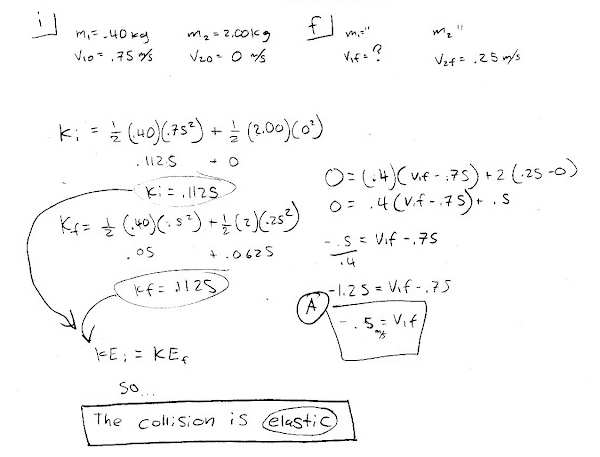A sample "t" response (from student 0361):Another sample "t" response (from student 1776):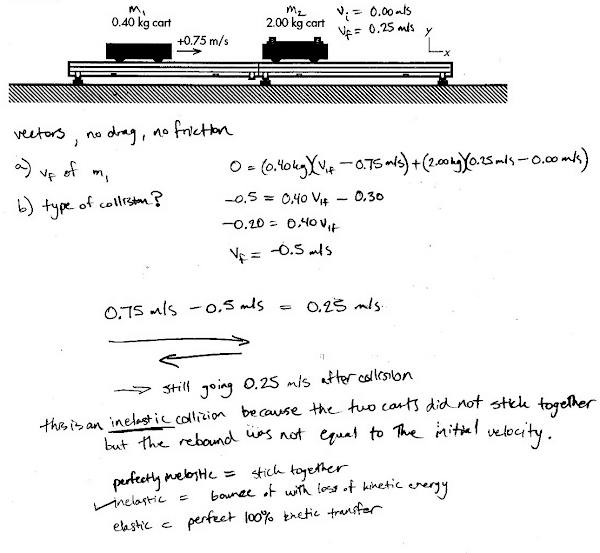Yet another sample "t" response (from student 1995):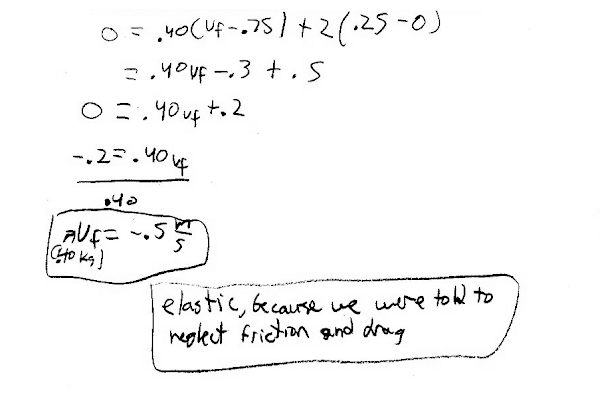## 20111218

### Physics final exam problem: disk rolling uphill

Physics 205A Final Exam, fall semester 2011
Cuesta College, San Luis Obispo, CA

Cf. Giambattista/Richardson/Richardson, Physics, 2/e, Problem 8.59, Review Exercise 10 (p. 311).A 0.45 kg solid disk of radius 0.050 m has an initial translational speed of 2.8 m/s as it rolls without slipping across a floor. The disk begins to roll up a ramp with a horizontal level 0.80 m above the floor. Determine whether the disk will make it to the horizontal level at the top of the ramp. Neglect drag. Show your work and explain your reasoning using the properties of rotational inertia, energy forms, and conservation of energy.

(Given: Idisk = (1/2)·M·R2.)

• p:
Correct. Sets up an energy conservation equation with changes in gravitational potential energy, translational kinetic energy, and rotational kinetic energy summing to zero (applying v = R·ω for rolling without slipping, and I = (1/2)·m·R2 for a solid cylinder), and solves for yi = 0.60 m, which is interpreted to mean that it will not reach the top of the ramp. Arithmetical
errors (± signs, cancellation, decimal point) okay.
• r:
Nearly correct, but includes minor math errors.
• t:
Nearly correct, but approach has conceptual errors, and/or major/compounded math errors.
• v:
Implementation of right ideas, but in an inconsistent, incomplete, or unorganized manner. Neglects rotational kinetic energy, thus solving for the translational speed of a sliding (point) mass. Or neglects translational kinetic energy, thus solving for the rotational speed of a cylinder given the gravitational potential energy of an equivalent point mass.
• x:
Implementation of ideas, but credit given for effort rather than merit. Garbled attempt at calculating energy terms, or may involve kinematics.
• y:
Irrelevant discussion/effectively blank.
• z:
Blank.

Sections 70854, 70855
Exam code: finalB0xs
p: 23 students
r: 0 students
t: 11 students
v: 7 students
x: 3 students
y: 0 students
z: 4 students

A sample "p" response (from student 3234):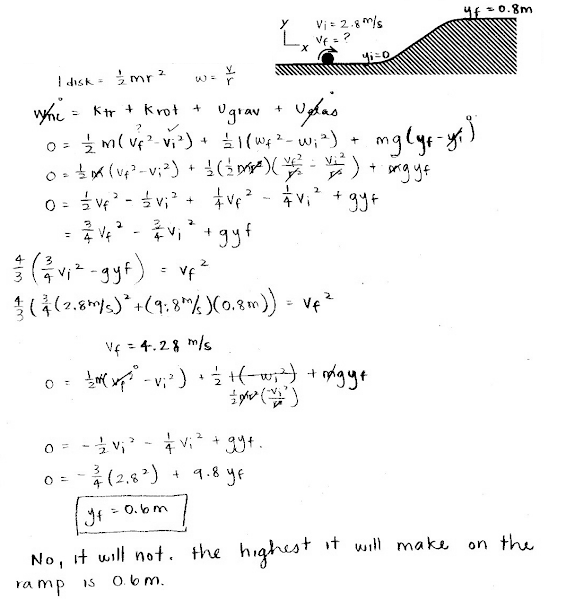Another sample "p" response (from student 4224):## 20111217

### Physics final exam problem: changing string tension and standing wave mode

Physics 205A Final Exam, fall semester 2011
Cuesta College, San Luis Obispo, CA

Cf. Giambattista/Richardson/Richardson, Physics, 2/e, Problem 11.49, Review & Synthesis 14 (p. 454).A string with a length of 0.80 m between supports is stretched by a 2.3 kg mass hanging from one end. A device oscillates the n = 3 mode at 84 Hz.      Find the mass that should hang off of this string such that the same 84 Hz frequency vibrates the n = 2 mode. Show your work and explain your reasoning using the properties of wave speeds, periodic waves, and standing waves.

• p:
Correct. Mass m of the string is not provided. However:

f3 = 84 Hz = ((3/(2·L))·sqrt(M·g/(m/L)),

where the hanging mass M = 2.3 kg, (m/L) is the linear mass density of the string; and:

f2' = 84 Hz = ((2/(2·L))·sqrt(Mg/(m/L)),

where M' is the new mass that hangs off of the string when vibrating in the n = 2 mode. Equating:

f2' = f3,

and cancelling common factors results in:

M' = M·(3/2)2 = 5.2 kg.

And/or may have solved for quantities such as wave speed along each string, linear mass density, etc., in intermediate steps.
• r:
Nearly correct, but includes minor math errors.
• t:
Nearly correct, but approach has conceptual errors, and/or major/compounded math errors. Typically calculates linear mass density (m/L) as (2.3 kg)/(0.80 m) = 5.6 kg/m, and/or confounds (m/L) with hanging mass M, tension force F with frequency f, etc., but still has systematic attempt at finding linear mass density from original n = 3 case, and then feeds (erroneous) (m/L) and/or other factors into the n = 2 case, along with other algebraic or nomenclature errors.
• v:
Implementation of right ideas, but in an inconsistent, incomplete, or unorganized manner. Some attempt at manipulating standing wave frequency equations.
• x:
Implementation of ideas, but credit given for effort rather than merit.
• y:
Irrelevant discussion/effectively blank.
• z:
Blank.

Sections 70854, 70855
Exam code: finalB0xs
p: 9 students
r: 5 students
t: 23 students
v: 6 students
x: 1 student
y: 2 students
z: 2 students

A sample "p" response (from student 1124):A sample "t" response (from student 3389):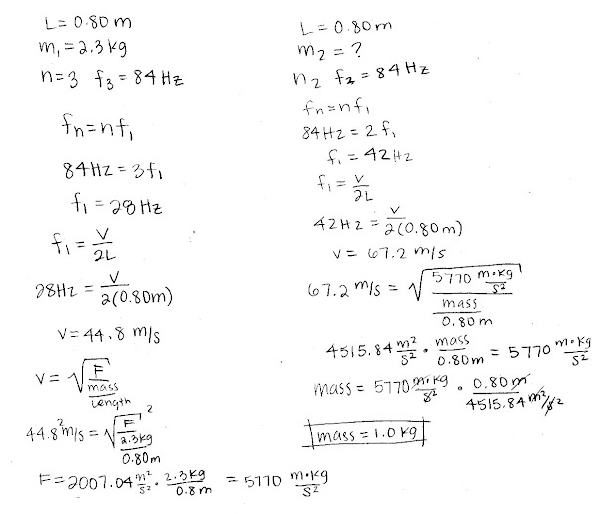Kudos (from student 0415):## 20111215

### Physics quiz question: thermal expansion of different cross-section bars

Physics 205A Quiz 7, fall semester 2011
Cuesta College, San Luis Obispo, CA

Cf. Giambattista/Richardson/Richardson, Physics, 2/e, Problem 13.9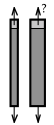A 1.0 cm2 cross-sectional area steel bar is heated up such that its length extends by by 0.1 mm. To extend a 2.0 cm2 cross-sectional area steel bar by 0.1 mm, it must experience a temperature change __________ the temperature change of the 1.0 cm2 cross-sectional area steel bar. (The bars have the same length.)
(A) smaller than.
(B) the same as.
(C) larger than.
(D) (Not enough information is given.)

Correct answer (highlight to unhide): (B)

The relative amount of linear expansion (∆L/L) is given by:

L/L = α·∆T,

such that the amount of expansion ∆L can be expressed as:

L = L·α·T,

and since ∆L does not depend on the cross-sectional area of the bar, then both bars of the same length L and same material α must experience the same temperature increase ∆T to expand by the same ∆L amount.

Sections 70854, 70855
Exam code: quiz07h3A7
(A) : 15 students
(B) : 20 students
(C) : 17 students
(D) : 0 students

Success level: 38%
Discrimination index (Aubrecht & Aubrecht, 1983): 0.71

### Physics quiz question: cooling metal container with liquid contents

Physics 205A Quiz 7, fall semester 2011
Cuesta College, San Luis Obispo, CA

Cf. Giambattista/Richardson/Richardson, Physics, 2/e, Problem 13.14

A metal container is filled to the brim with a liquid. If the temperature decreases, under which conditions would the liquid overflow? (There are no changes in phase.)
(A) 3αmetal > βliquid.
(B) 3αmetal = βliquid.
(C) 3αmetal < βliquid.
(D) (Two of the above choices.)
(E) (All of the above choices.)
(F) (None of the above choices.)

Correct answer (highlight to unhide): (A)

If the temperature decreases, the liquid would overflow the container if the metal container contracted more than the liquid contents. This means that the volume expansion coefficient of the metal would need to be greater than the volume expansion coefficient of the liquid.

Sections 70854, 70855
Exam code: quiz07h3A7
(A) : 29 students
(B) : 1 student
(C) : 17 students
(D) : 5 students
(E) : 0 students
(F) : 0 students

Success level: 56%
Discrimination index (Aubrecht & Aubrecht, 1983): 0.76

### Physics quiz question: heat flow rate through window and shade

Physics 205A Quiz 7, fall semester 2011
Cuesta College, San Luis Obispo, CA

Cf. Giambattista/Richardson/Richardson, Physics, 2/e, Problem 14.56Hunter Douglas

A Harvey Building Products vinyl casement window [*] has a thermal resistance of 0.33 K/watt. The temperature difference between inside and outside is 30° C. The SymphonyShades™ Virtuoso® window shade[**] is also claimed to have a thermal resistance of approximately 0.33 K/watt. If this window shade completely covers the window, the rate of heat conduction per time will be __________ of the original (uncovered) value.
(A) one-third.
(B) one-half.
(C) two-thirds.
(D) twice.
(E) (No change in rate of heat conduction.)

[*] R-factor = 0.33, ENERGY STAR rated, 19 square feet, harveybp.com/upload/products/literature/Harvey_AccessoryWindows_Brochure.pdf

The rate at which heat is conducted per time to the environment through the window given by:

Powerwindow = ((heat flow)/time)window = ∆T/Rwindow,

where Rwindow is the thermal resistance of the window:

Rwindow = dwindow/(κwindow·Awindow),

which is already given as 0.33 K/watt, such that the heat flow per time conducted through the uncovered window is:

Powerwindow = (30° C)/(0.33 K/watt) = 90.909090 watts.

Thermal resistances are additive for materials that are stacked in series, so the heat flow per time through the window and shade will be:

Powerwindow & shade = (30° C)/(0.33 K/watt + 0.33 K/watt) = 45.45454545 watts.

Thus increasing the overall thermal resistance by a factor of two will reduce the heat flow per time to one-half of its original (uncovered) value.

Sections 70854, 70855
Exam code: quiz07h3A7
(A) : 9 students
(B) : 27 students
(C) : 8 students
(D) : 7 students
(E) : 1 student

Success level: 52%
Discrimination index (Aubrecht & Aubrecht, 1983): 0.67

### Physics quiz question: net heat rate radiated by stove

Physics 205A Quiz 7, fall semester 2011
Cuesta College, San Luis Obispo, CA

Cf. Giambattista/Richardson/Richardson, Physics, 2/e, Problem 14.65

A black wood stove has a surface area of 1.6 m2 and a surface temperature of 180° C. The room temperature is 15° C. What is the net rate at which heat is radiated into the room?
(A) 67 watts.
(B) 95 watts.
(C) 3.2x103￼ watts.
(D) 3.8￼x103 watts.

The rate at which the wood stove radiates heat to the environment is given by:

(heat flow)/time = e*σ*A*(Tobj)4 = 3,820 watts,

which is response (D), where the temperature of the object is given in kelvins: 180° C + 273 = 453 K.

The rate at which the wood stove absorbs heat from the environment is given by:

(heat flow)/time = e*σ*A*(Tenv)4 = 624 watts,

where the temperature of the environment is given in kelvins: 15° C + 273 = 288 K.

Thus the net rate at which heat is radiated into the environment is then:

(heat flow)/time = e*σ*A*[(Tobj)4 - (Tenv)4] = 3,196 watts,

or to two significant figures, 3.2x103 watts.

Response (A) uses the temperature difference raised to the fourth power (Tobj - Tenv)4; response (B) is the power radiated by the stove, but using 180° C instead of 453 K.

Sections 70854, 70855
Exam code: quiz07h3A7
(A) : 3 students
(B) : 24 students
(C) : 23 students
(D) : 2 students

Success level: 44%
Discrimination index (Aubrecht & Aubrecht, 1983): 0.46

### Physics quiz question: lava lamp convection

Physics 205A Quiz 7, fall semester 2011
Cuesta College, San Luis Obispo, CA

Cf. Giambattista/Richardson/Richardson, Physics, 2/e, Conceptual Question 14.8

Wax droplets in a lava lamp will rise after being heated in the base because their __________ increases, making their __________ decrease.
(A) density; mass.
(B) density; volume.
(C) mass; volume.
(D) mass; density.
(E) volume; mass.
(F) volume; density.

The light bulb at the base of the lava lamp heats wax suspended in fluid, which causes the wax to expand and increase in volume, decreasing in density. The expanded wax then rises, as it is now less dense than the fluid it is suspended in. Later after cooling at the top of the lamp, the wax will contract, decrease in volume, increasing in density, and subsequently sink.

Sections 70854, 70855
Exam code: quiz07h3A7
(A) : 1 student
(B) : 1 student
(C) : 0 students
(D) : 0 students
(E) : 0 students
(F) : 50 students

Success level: 96%
Discrimination index (Aubrecht & Aubrecht, 1983): 0.12

## 20111214

### Physics quiz archive: temperature, thermal equilibrium, heat transfer

Physics 205A Quiz 7, fall semester 2011
Cuesta College, San Luis Obispo, CA

Sections 70854, 70855, version 1
Exam code: quiz07n0N3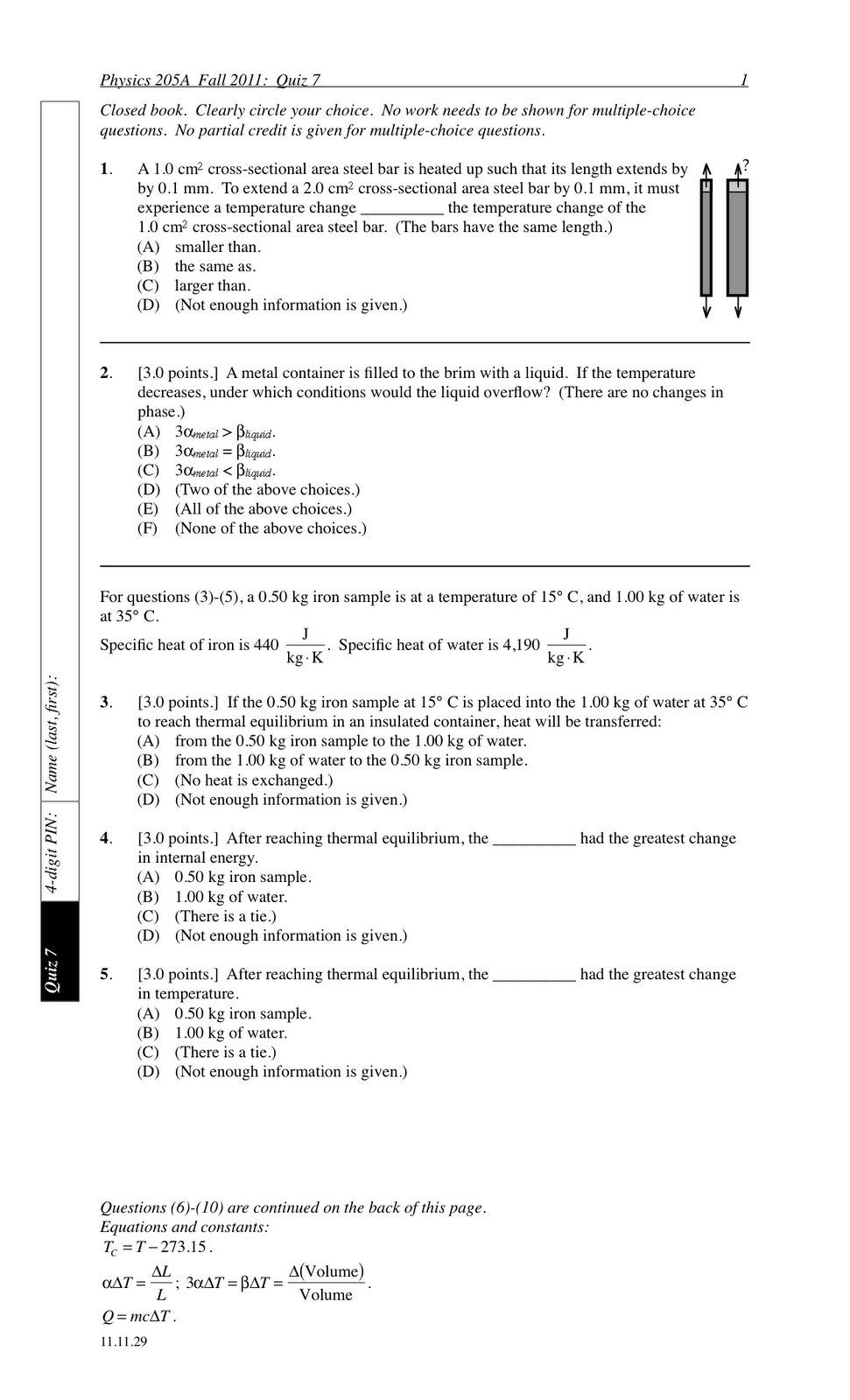Sections 70854, 70855 results
Quiz 7 results (max score = 30):
 0- 6 : * [low = 6] 7-12 : ******** 13-18 : ******************* 19-24 : *************** [mean = 19.2 +/- 5.7] 25-30 : ********* [high = 30]

## 20111213

### Astronomy multiple-choice question: solar system debris and IAU classification scheme

Astronomy 210 Quiz 7, fall semester 2011
Cuesta College, San Luis Obispo, CA

Refer below to the minimal qualifications established by the International Astronomical Union for a planet:
I. Orbits the sun.
II. Shape "rounded-out" by gravity.
III. Cleared/dominates orbit around sun.
Which of the following is classified as solar system debris?
(A) Pluto, a spherical body sharing Neptune's orbit.
(B) Ceres, a spherical body with an orbit between Mars and Jupiter.
(C) Cruithne, an irregularly shaped body sharing Earth's orbit.
(D) Phobos, an irregularly shaped body orbiting Mars.
(E) (More than one of the above choices.)
(F) (All of the above choices.)
(G) (None of the above choices.)

Solar system debris are objects that orbit the sun (meets qualification I), but are irregular in shape (does not meet qualification II), and also are unable to dominate/clear its orbit around the sun (does not meet qualification III). Thus according to the IAU classification scheme, Cruithne is the only solar system debris object in this list, as Pluto and Ceres are dwarf planets, and Phobos is a satellite.

Section 70158
Exam code: quiz07s3tI
(A) : 1 student
(B) : 2 students
(C) : 7 students
(D) : 4 students
(E) : 15 students
(F) : 1 student
(G) : 1 student

Success level: 30% (including partial credit for multiple-choice)
Discrimination index (Aubrecht & Aubrecht, 1983): 0.60

Section 70160
Exam code: quiz07n0N3
(A) : 1 student
(B) : 2 students
(C) : 9 students
(D) : 2 students
(E) : 9 students
(F) : 4 students
(G) : 1 student

Success level: 40% (including partial credit for multiple-choice)
Discrimination index (Aubrecht & Aubrecht, 1983): 0.75

## 20111212

### Astronomy multiple-choice question: Amalthea and IAU classification scheme

Astronomy 210 Quiz 7, fall semester 2011
Cuesta College, San Luis Obispo, CA

Refer below to the minimal qualifications established by the International Astronomical Union for a planet:
I. Orbits the sun.
II. Shape "rounded-out" by gravity.
III. Cleared/dominates orbit around sun.
Amalthea, an asteroid, was captured by Jupiter and became its satellite. Which qualification(s) changed when this happened?
(A) I only.
(B) II only.
(C) III only.
(D) Both I and II.
(E) Both II and III.
(F) Both I and III.
(G) I, II, and III.
(H) (None of the above choices.)

Amalthea, while an asteroid, orbited the sun (met qualification I), but did not clear/dominate its orbit around the sun in the asteroid belt (did not meet qualification III). When captured by Jupiter to become its satellite, qualification I changed, as now it no longer orbits the sun. Even though the shape of Amalthea was not discussed, it would not have changed when captured by Jupiter.

Section 70158
Exam code: quiz07s3tI
(A) : 9 students
(B) : 2 students
(C) : 6 students
(D) : 4 students
(E) : 2 students
(F) : 2 students
(G) : 2 students
(H) : 4 students

Success level: 35% (including partial credit for multiple-choice)
Discrimination index (Aubrecht & Aubrecht, 1983): 0.48

Section 70160
Exam code: quiz07n0N3
(A) : 15 students
(B) : 4 students
(C) : 3 students
(D) : 2 students
(E) : 0 students
(F) : 3 students
(G) : 0 students
(H) : 1 student

Success level: 57% (including partial credit for multiple-choice)
Discrimination index (Aubrecht & Aubrecht, 1983): 0.38

## 20111211

### Astronomy quiz archive: solar system

Astronomy 210 Quiz 7, fall semester 2011
Cuesta College, San Luis Obispo, CA

Section 70160, version 1
Exam code: quiz07n0N3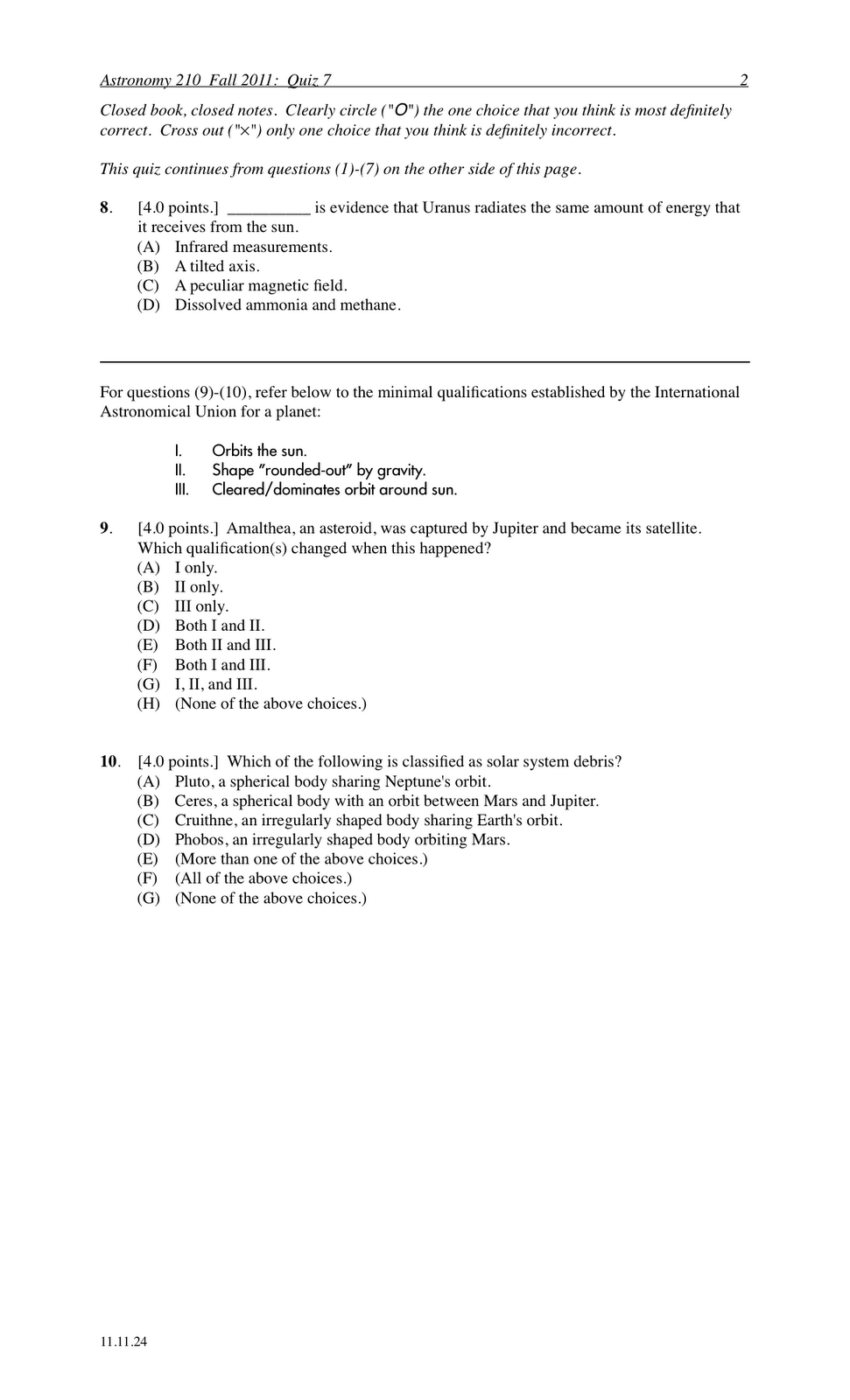Section 70160
Quiz 7 results (max score = 40):
 0- 8.0 : 8.5-16.0 : ***** [low = 11.5] 16.5-24.0 : ********** 24.5-32.0 : ******** [mean = 24.5 +/- 8.1] 32.5-40.0 : ***** [high = 40.0]

### Astronomy quiz archive: solar system

Astronomy 210 Quiz 7, fall semester 2011
Cuesta College, San Luis Obispo, CA

Section 70158, version 1
Exam code: quiz07s3tI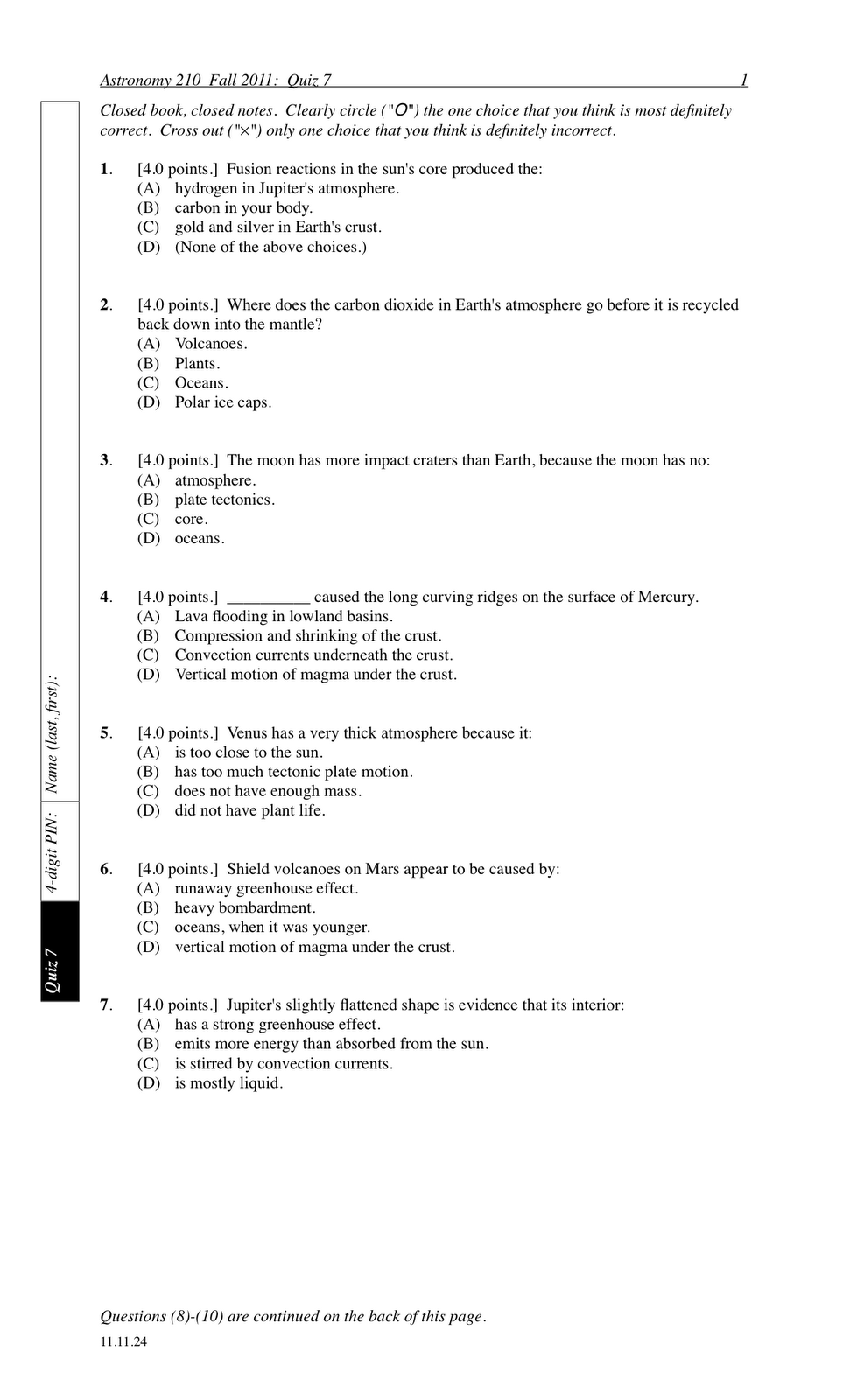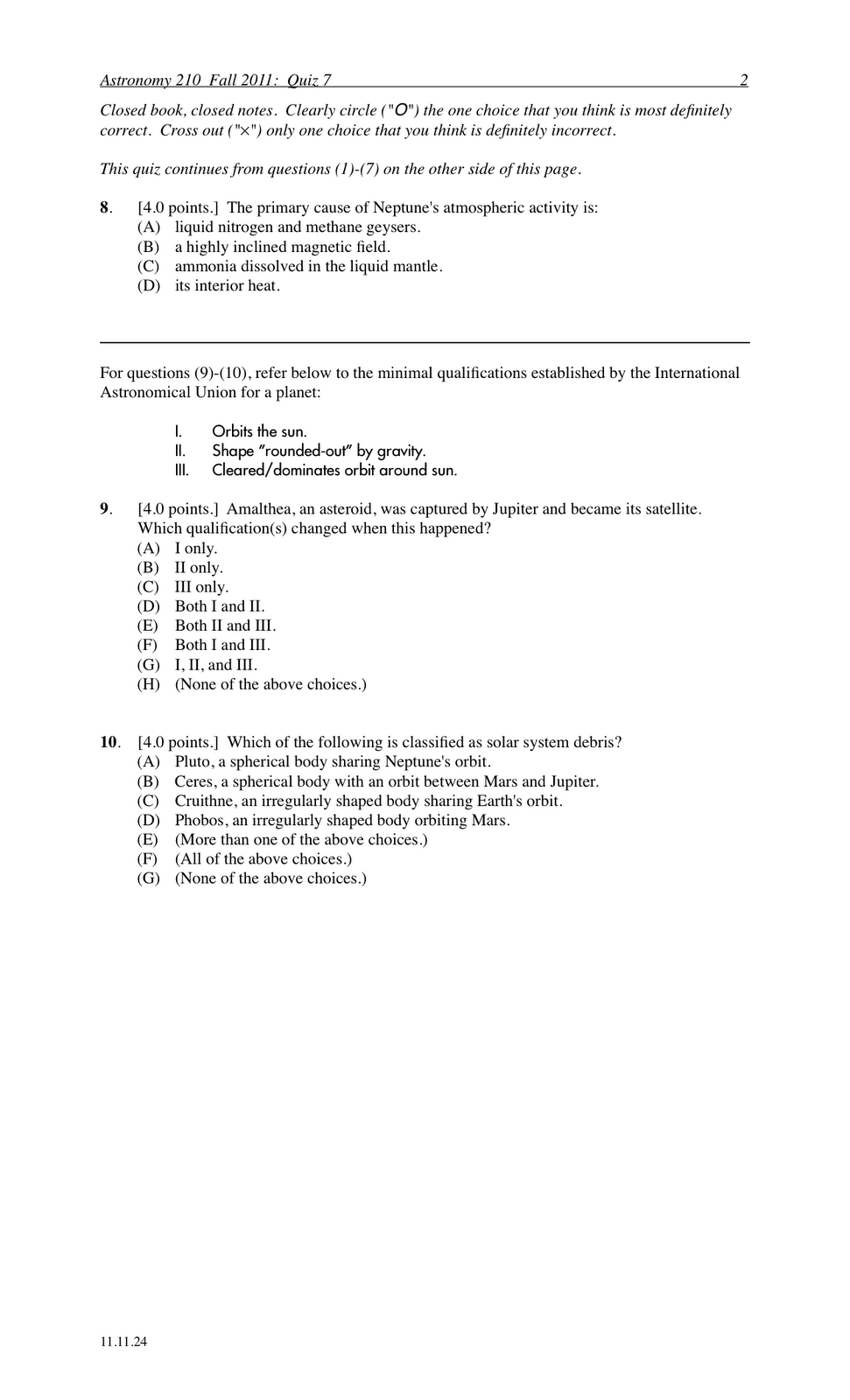Section 70158
Quiz 7 results (max score = 40):
 0- 8.0 : ** [low = 4.0] 8.5-16.0 : ******* 16.5-24.0 : ***************** [mean = 18.8 +/- 7.2] 24.5-32.0 : **** 32.5-40.0 : * [high = 40.0]

## 20111210

### Total lunar eclipse, December 10, 2011111210-1280484-1
http://www.flickr.com/photos/waiferx/6486545953/

Wifey X and Briquetta (puppy) watching the total lunar eclipse, December 10, 2011.

## 20111207

### FCI post-test comparison: Cuesta College versus UC-Davis (fall semester 2011)

Students at both Cuesta College (San Luis Obispo, CA) and the University of California at Davis were administered the 30-question Force Concept Inventory (Doug Hestenes, et al.) during the last week of instruction.

 Cuesta CollegePhysics 205AFall semester 2011 UC-DavisPhysics 7BSummer session II 2002 N 46 students* 76 students* low 6 3 mean 15.2 ± 6.7 12.9 ± 5.5 high 30 26

*Excludes students with negative informed consent forms (*.pdf)

Student's t-test of the null hypothesis results in p = 0.041 (t = 2.06, sdev = 5.96, degrees of freedom = 120), thus there is a significant difference between Cuesta College and UC-Davis FCI post-test scores.

The pre- to post-test gain for this semester at Cuesta College is:

 Physics 205A fall semester 2011 sections 70854, 7085 = 31% ±17% (N = 57) = 51% ± 23% (N = 46) = 0.28 ± 0.29 (matched-pairs); 0.28 (class-wise)

This Hake gain is in line with the previous semesters' results for algebra-based introductory physics at Cuesta College (0.25-0.33), and greater than previous gains for algebra-based introductory physics at UC-Davis (0.16), and for calculus-based introductory physics at Cuesta College (0.14-0.16), as discussed in previous postings on this blog.

Notable about this Physics 205A class at Cuesta College during this fall 2011 semester is the use of flashcards rather than electronic response system "clickers" (Classroom Performance System, einstruction.com), to engage in "think-pair-share" (peer-instruction).

D. Hestenes, M. Wells, and G. Swackhamer, Arizona State University, "Force Concept Inventory," Phys. Teach. 30, 141-158 (1992).
Development of the FCI, a 30-question survey of basic Newtonian mechanics concepts.

Previous FCI results:

## 20111205

### Overheard: all that glitters

Astronomy 210L, fall semester 2011
Cuesta College, San Luis Obispo, CA

(Overheard while students set up their research posters for the last astronomy lab meeting.)

Student: "Do you like how I did the galaxies [at the top of the poster]?"

Instructor: "Are those [elliptical galaxy and spiral galaxy] just glitter and glue?"

Student: "Yeah. I had some space to fill in so I went with that."

Instructor: "Seriously, when was the last time you got to use glitter and glue?"

Student: (Beat.) "I'll have to get back to you on that."

## 20111204

### Overheard: fabulous graph

Astronomy 210L, fall semester 2011
Cuesta College, San Luis Obispo, CA

(Overheard while students set up their research posters for the last astronomy lab meeting.)

Instructor: "Are these data points on your graph...sequins?"

Student: "No, they're just drawn in with purple ink."

Instructor: "That's too bad." (Beat.) "Because if they were sequins, your graph would be...fabulous!"

## 20111202

### Astronomy current events question: early pristine gas

Astronomy 210L, fall semester 2011
Cuesta College, San Luis Obispo, CA

Students are assigned to read online articles on current astronomy events, and take a short current events quiz during the first 10 minutes of lab. (This motivates students to show up promptly to lab, as the time cut-off for the quiz is strictly enforced!)
John Matson, "Fresh Start: Scientists Glimpse Unsullied Traces of the Infant Universe," November 10, 2011
http://www.scientificamerican.com/article.cfm?id=big-bang-nucleosynthesis-gas
Pockets of pristine gas from the early universe were detected to have no heavy elements by observing __________ the gas clouds.
(A) no dark matter within.
(B) no massive black holes in.
(C) the gravitational redshift of.
(D) first-generation stars being born in.
(E) how quasar light passes through.

Student responses
Sections 70178, 70186, 70200
(A) : 5 students
(B) : 1 student
(C) : 9 students
(D) : 11 students
(E) : 20 students

### Astronomy current events question: Pluto's hidden ocean?

Astronomy 210L, fall semester 2011
Cuesta College, San Luis Obispo, CA

Students are assigned to read online articles on current astronomy events, and take a short current events quiz during the first 10 minutes of lab. (This motivates students to show up promptly to lab, as the time cut-off for the quiz is strictly enforced!)
Nola Taylor Redd, "Pluto's Hidden Ocean," November 17, 2011
http://www.astrobio.net/exclusive/4343/plutos-hidden-ocean-
When NASA's New Horizons spacecraft passes by Pluto in 2015, it is expected to verify whether or not there is a subsurface ocean by looking at Pluto's:
(A) surface contours.
(B) geyser outflow composition.
(C) habitable zone.
(D) crater density.
(E) atmosphere composition.

Student responses
Sections 70178, 70186, 70200
(A) : 14 students
(B) : 3 students
(C) : 2 students
(D) : 2 students
(E) : 3 students

### Astronomy current events question: Europa's 'Great Lake?'

Astronomy 210L, fall semester 2011
Cuesta College, San Luis Obispo, CA

Students are assigned to read online articles on current astronomy events, and take a short current events quiz during the first 10 minutes of lab. (This motivates students to show up promptly to lab, as the time cut-off for the quiz is strictly enforced!)
UTC-Austin news release, "Scientists Find Evidence for 'Great Lake' on Europa and Potential New Habitat for Life," November 16, 2011
http://www.jsg.utexas.edu/news/2011/11/scientists-find-evidence-for-great-lake-on-europa/
Based on __________, University of Texas at Austin scientists have proposed a model predicting lakes within the icy shell of Jupiter's moon, Europa.
(B) reflected light patterns.
(C) photosynthesis chemical byproducts.
(D) drilling down into the ice sheet in Antarctica.
(E) comparing images with similar features on Earth.

Student responses
Sections 70178, 70186, 70200
(A) : 8 students
(B) : 5 students
(C) : 2 students
(D) : 1 student
(E) : 6 students

### Astronomy current events question: matter-antimatter decay imbalance

Astronomy 210L, fall semester 2011
Cuesta College, San Luis Obispo, CA

Students are assigned to read online articles on current astronomy events, and take a short current events quiz during the first 10 minutes of lab. (This motivates students to show up promptly to lab, as the time cut-off for the quiz is strictly enforced!)
Jason Palmer, "LHC Reveals Hints of 'New Physics' in Particle Decays," November 15, 2011
http://www.bbc.co.uk/news/science-environment-15734668
Preliminary observations of __________ at the Large Hadron Collider may explain why the universe is predominately matter.
(A) different particle and antiparticle decay rates.
(B) neutrinos traveling faster than the speed of light.
(C) dark matter and dark energy production.
(D) miniature short-lived black holes.
(E) lack of time-reversed big banglets.

Student responses
Sections 70178, 70186, 70200
(A) : 30 students
(B) : 4 students
(C) : 10 students
(D) : 2 students
(E) : 0 students

### Physics midterm question: work done on diagonally-pulled crate

Physics 205A Midterm 2, fall semester 2011
Cuesta College, San Luis Obispo, CA

Cf. Giambattista/Richardson/Richardson, Physics, 2/e, Problem 6.1A rope that makes an angle of 35° above the horizontal drags a crate to the right across a frictionless floor. The __________ force does positive work on the crate.
(A) weight.
(B) rope tension.
(C) normal.
(D) (More than one of the above choices.)
(E) (None of the above choices.)

Correct answer (highlight to unhide): (B)

The definition of work done is:

W = F·s·cosθ,

and since the angle between the displacement s (along the +x direction) is 90° for both the normal force and the weight force, these forces do zero work on the crate. Since the angle between the tension force of the rope and the displacement s is between 0° and 90°, then the rope does positive work on the crate.

Sections 70854, 70855
Exam code: midterm02fR3q
(A) : 1 student
(B) : 30 students
(C) : 0 students
(D) : 19 students
(E) : 3 students

Success level: 57%
Discrimination index (Aubrecht & Aubrecht, 1983): 0.22

### Physics midterm question: horizontal, widening pipe

Physics 205A Midterm 2, fall semester 2011
Cuesta College, San Luis Obispo, CA

Cf. Giambattista/Richardson/Richardson, Physics, 2/e, Problem 9.41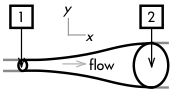The pressure of water __________ as it flows from point  to point . (Assume ideal fluid flow.)
(A) decreases.
(B) remains constant.
(C) increases.
(D) (Not enough information is given.)

From applying the continuity equation:

A1·v1 = A2·v2,

the water speed at point  is slower than at point , because the cross-sectional area at point  is larger than at point .

Then from Bernoulli's equation:

0 = ∆P + (1/2)·ρ·∆(v2) + ρ·g·∆y,

the (1/2)·ρ·∆(v2) term on the right-hand side of the equation decreases due to the decrease in speed from point  to point , while the ρ·g·∆y term remains constant (no change in elevation along the horizontal pipe), thus the pressure must increase as water flows from point  to point .

Sections 70854, 70855
Exam code: midterm02fR3q
(A) : 24 students
(B) : 14 students
(C) : 15 students
(D) : 0 students

Success level: 28%
Discrimination index (Aubrecht & Aubrecht, 1983): 0.43

### Physics midterm question: piccolo versus flute

Physics 205A Midterm 2, fall semester 2011
Cuesta College, San Luis Obispo, CA

Cf. Giambattista/Richardson/Richardson, Physics, 2/e, Conceptual Questions 12.12, Problem 12.19Piccolos and flutes can both be modeled as open-open pipes. If a piccolo is one-half the length of a flute, the fundamental frequency of the piccolo is __________ the fundamental frequency of a flute.
(A) one-quarter.
(B) one-half.
(C) the same as.
(D) twice.
(E) four times.

The fundamental frequency of a standing sound wave in an open-open pipe is given by:

f1 = v/(2*L).

Since the piccolo is half the length of the flute, then it will have a fundamental frequency twice that of the flute.

Sections 70854, 70855
Exam code: midterm02fR3q
(A) : 2 students
(B) : 7 students
(C) : 1 student
(D) : 41 students
(E) : 2 students

Success level: 77%
Discrimination index (Aubrecht & Aubrecht, 1983): 0.27

### Physics midterm question: standing wave in warm closed-open pipe

Physics 205A Midterm 2, fall semester 2011
Cuesta College, San Luis Obispo, CA

Cf. Giambattista/Richardson/Richardson, Physics, 2/e, Conceptual Questions 12.1, 12.2, Problem 12.21

If the temperature of the air inside a closed-open pipe were to heat up, the speed of sound in the pipe would __________, while its fundamental frequency would __________.
(A) remain constant; remain constant.
(B) remain constant; change.
(C) change; remain constant.
(D) change; change.

Correct answer (highlight to unhide): (D)

The fundamental frequency of a standing sound wave in a closed-open pipe is given by:

f1 = v/(4·L),

and the speed of sound waves is given by:

v = (331 m/s)·sqrt(T/(273 K)),

such that a temperature increase would increase the speed of sound, resulting in a higher fundamental frequency. (While the frequency of a sound wave already resonating in the pipe would not be affected by the change in speed due to the increase in temperature, this existing sound wave frequency would no longer be able to cause resonance in the pipe due to the change in the pipe's fundamental frequency requirement.)

Sections 70854, 70855
Exam code: midterm02fR3q
(A) : 7 students
(B) : 7 students
(C) : 9 students
(D) : 30 students

Success level: 55%
Discrimination index (Aubrecht & Aubrecht, 1983): 0.22

### Physics midterm question: decreasing the fundamental frequency

Physics 205A Midterm 2, fall semester 2011
Cuesta College, San Luis Obispo, CA

Cf. Giambattista/Richardson/Richardson, Physics, 2/e, Conceptual Question 11.4, Multiple-Choice Question 11.5

A cello's C-string[*],[**] has a length of 0.70 m and is stretched to a tension of 140 N. It vibrates at a fundamental frequency of 65.4 Hz. __________ would decrease the fundamental frequency of this cello string.
(A) Pressing a finger on the string, such that a shorter length of it vibrates.
(B) Increasing the tension of the string.
(C) (Either of the above choices.)
(D) (Neither of the above choices.)

[**] rdebey.com/string_tension.htm.

Correct answer (highlight to unhide): (D)

The speed of waves along the string is given by:

v = √(F/(m/L)).

Using a shorter length of string would not change the tension F, and would neither change the linear mass density (m/L)--while L would decrease, the mass m would also proportionally decrease as well, keeping the ratio (m/L) constant. Thus the speed v would remain unchanged.

The fundamental frequency of a standing wave on a string is given by:

f1 = v/(2·L).

Then using a shorter length of string would increase the fundamental frequency f1 (as the speed v would remain constant).

As for increasing the tension in the string, since:

v = √(F/(m/L)),

the linear mass density (m/L) would remain constant, such that the speed v would increase. Then for the fundamental frequency of a standing wave on a string:

f1 = v/(2·L),

increasing the speed v would then increase the fundamental frequency f1 (as L would remain constant).

Thus neither pressing a finger on the string (such that a shorter length of it vibrates), nor increasing the tension of the string would decrease the fundamental frequency f1.

Sections 70854, 70855
Exam code: midterm02fR3q
(A) : 7 students
(B) : 7 students
(C) : 9 students
(D) : 30 students

Success level: 55%
Discrimination index (Aubrecht & Aubrecht, 1983): 0.22

## 20111201

### Physics midterm question: cable-supported beam

Physics 205A Midterm 2, fall semester 2011
Cuesta College, San Luis Obispo, CA

Cf. Giambattista/Richardson/Richardson, Physics, 2/e, Problems 8.19, 8.35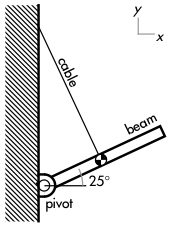A uniform beam is mounted on a pivot at one end, and supported at its center by a cable perpendicular to the beam. Discuss why the magnitude of the tension in the cable is less than the weight of the beam. Explain your reasoning using diagram(s) with locations of forces and perpendicular lever arms, the properties of torques, and Newton's laws.

• p:
Correct. The counterclockwise torque from cable equals the clockwise torque from weight, but the cable has a larger lever arm (L/2) than weight (L·cos(25°)/2), such that the cable tension force must be smaller than the weight force.
• r:
As (p), but argument indirectly, weakly, or only by definition supports the statement to be proven, or has minor inconsistencies or loopholes.
• t:
Nearly correct, but argument has conceptual errors, or is incomplete. Applies Newton's first law for rotations, but r discussion is garbled or missing.
• v:
Limited relevant discussion of supporting evidence of at least some merit, but in an inconsistent or unclear manner. At least attempts to discuss torques, forces, lever arms, but Newton's first law for rotations is not clearly used. May instead use Newton's first law for vertical forces and involve the force of the pivot point, but does not clearly break up tension into vertical and horizontal components.
• x:
Implementation of ideas, but credit given for effort rather than merit. Does not discuss torques, forces, lever arms.
• y:
Irrelevant discussion/effectively blank.
• z:
Blank.

Sections 70854, 70855
Exam code: midterm02fR3q
p: 16 students
r: 3 students
t: 10 students
v: 24 students
x: 0 students
y: 0 students
z: 0 students

A sample "p" response (from student 4816):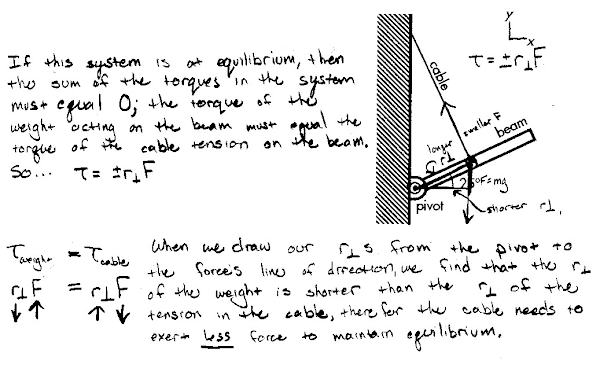### Physics midterm question: crushing-fender car

Physics 205A Midterm 2, fall semester 2011
Cuesta College, San Luis Obispo, CA

Cf. Giambattista/Richardson/Richardson, Physics, 2/e, Problem 7.13

A car is retrofitted with special fenders that would completely crush on impact, such that it would come to a complete stop (and not rebound) after crashing into the wall. Discuss why this retrofitted car would experience a smaller magnitude crash impulse than a car that would rebound slightly after crashing into a wall. (Assume that the addition of special fenders do not substantively change the mass of the car.) Explain your reasoning using the properties of impulse and momentum.

• p:
Correct. Since impulse is the change in momentum, discusses how the retrofitted car undergoes a smaller momentum change than a car that rebounds off of the wall.
• r:
As (p), but argument indirectly, weakly, or only by definition supports the statement to be proven, or has minor inconsistencies or loopholes. May have slightly garbled definition of impulse-momentum theorem, but argument is conceptually sound.
• t:
Nearly correct, but argument has conceptual errors, or is incomplete. At least understands that ∆v magnitude is greater for rebounding car in analyzing the car crashes with regards to momentum change.
• v:
Limited relevant discussion of supporting evidence of at least some merit, but in an inconsistent or unclear manner. Some attempt at applying impulse and momentum concepts, but may have involved force and/or elapsed time in argument.
• x:
Implementation of ideas, but credit given for effort rather than merit. Discussion based on how momentum or kinetic energy is "absorbed" or "transmitted back" by crushing or rebounding fenders.
• y:
Irrelevant discussion/effectively blank.
• z:
Blank.

A sample "p" response (from student 0524):